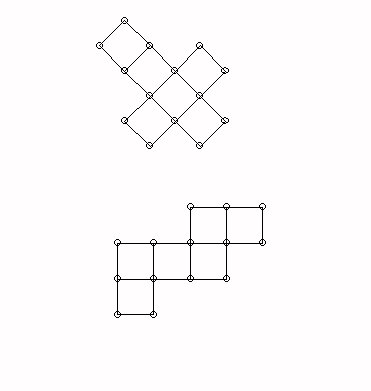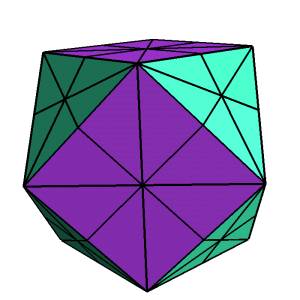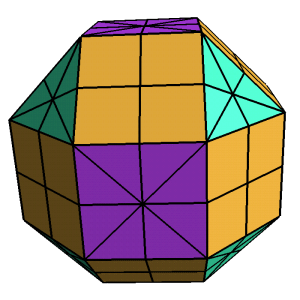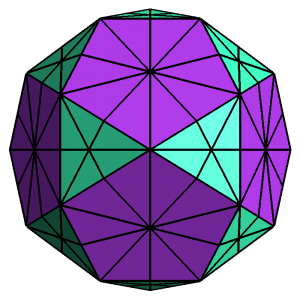Tuesday,  February 24
Comment on Project  Proposals  and  portfolio sample entries.
Review of Discussion So Far on Classification of Isometries
Video : Isometries
The video introduced the four isometries we have discussed:
reflections, rotations, translations, and glide reflections.

It showed that the product of two reflections is either a rotation (if the axes of the reflection intersect)  or a translation (if the axes of the reflection are parallel).

We saw this also with a Wingeometry demonstration for reflection- one and two reflections

he video showed that
Any plane isometry  is either a reflection or  the product of two or three reflections.
Two reflections = rotation or translation.

Three reflections = reflection or glide reflection
Preview of visual Proof: Key idea- The product of two reflections is "flexible."

How to figure out what isometry you have.... match features.

 Preserve Orientation Reverse Orientation No Fixed points Translation Glide reflection Fixed Point(s) Rotation Reflection

Using Isometries to create variations of tilings
Kali: Symmetry group

• 180 degree Rotations
• Activity for modifying tilings
• Translations

• Comment on Symmetry  in Music and Sound:
we discussed
Translation   .... by an octave, relative position-- chords
Reflection
Rotation: thirteen notes in "chromatic" scale
Glide Reflection

Space: How do we understand objects in space?
How can the Flatlander experience the sphere and space?

Try making a torus with 2 and 1 piece!

Cross sections: Look at the cube with cross sections : squares, rectangles, triangles and hexagons depending on how the square passes through the plane.

Shadows: Look at how the cube might case shadows that were square, rectangular or hexagonal,

Fold downs- flattened figures: Consider how the cube can be assembled from folded down squares in two different configurations: a cross or a "zig-zag."analogue...  point... line.... polygon.... polyhedron......
• Some Issues we'll consider in space:
• Polyhedra and symmetry.
• Historical Note on Kepler.
• Platonic (regular convex polyhedra) and Archimedean (semiregular convex) Solids- on the web!
• The 5 Platonic Solids

Octahedron   Tetrahedron   Icosahedron
Cube                                  Dodecahedron
• Why are there only 5?
• Look at the possible ways to put a single regular polygon together with more of the same to make a spatial "cap" about a single vertex. This involves equilateral triangles (3,4, or 5), squares (3) or pentagons (3).
• This shows that there were at most five vertex caps possible. These actually do work to make
• Regular polygons around a vertex.
• All vertices are "the same"
• Activity: Counting vertices, edges, and faces for the platonic solids to become more familiar with them.
•  The 13 Archimedean Solids

• Symmetries (Isometries) in the plane compared to those in space- an introduction:
• Spatial Symmetry
The Platonic and Archimedean Solids
.CubeoctahedronRhombicubeoctahedronIcosidodecahedron
• Translations
• Rotations: Center point - central axis
• Reflection :  across line - across plane
• Symmetries of the cube:
• Rotations
• reflections
• rotation- reflection
• Isometries in space: products of reflections in space:
• Rotations and translations
• Applications to dance